## 让我们来创建后期效果-高动态光照渲染!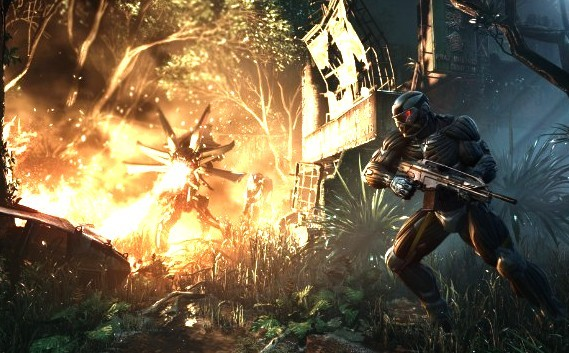[cc lang=”C++”]

kernel Lum
< namespace : "cn.dreamfairy"; vendor : "dreamfairy"; version : 1; >
{
input image4 baseTex;
input image4 bloomTex;
output pixel4 dsts;

//外部参数
parameter float fAvgLum
< minValue:float(0.1); maxValue:float(0.5); defaultValue:float(0.5); >;

parameter float fDimmer
< minValue:float(0); maxValue:float(1); defaultValue:float(1); >;

void
evaluatePixel()
{
float4 texCol = sampleNearest(baseTex,outCoord());
//计算平均亮度
float vLum = 0.27 * texCol.r + 0.67 * texCol.g + 0.06 * texCol.b;
//亮度缩放
float vLumScaled = fDimmer * vLum / fAvgLum;

texCol = float4(texCol.r * vLumScaled, texCol.g * vLumScaled,
texCol.b * vLumScaled, texCol.a * vLumScaled);
texCol = texCol / (float4(1.0) + texCol);

float4 texBloom = sampleNearest(bloomTex, outCoord());
dsts = texBloom + texCol;
}
}
[/cc]

## 让我们来创建后期效果-模糊!

var index : uint = step <= 1 ? 1 : (step - 1) * 3; var num : uint = 8 * index; 之后我们需要用拼接的方式来构造AGAL [cc lang="actionscript3"] private function getBlurAgal(step : uint, force : uint, coord : String, pTempIndex : int, pSaveIndex : int, pConstantOffset : int, pTextureIndex : int, constant : Vector., pInitIndex : int) : String
{
var data : Array = new Array();
var isFirstSave : Boolean = true;
var loopNum : uint = 0;
var stepX : Number = force / stage.stageWidth;
var stepY : Number = force / stage.stageHeight;
var index : uint = step <= 1 ? 1 : (step - 1) * 3; var num : uint = 8 * index; var tempIndex : int = pTempIndex; var saveIndex : int = pSaveIndex; var textureIndex : int = pTextureIndex; var initIndex : int = pInitIndex; var constantOffset : int = pConstantOffset; constant = constant || new Vector.();
constant.length = 0;

data.push(“mov ft” + tempIndex + “, fc” + initIndex);

//横向遍历
for(var i : int = -step; i <= step; i++) { //纵向遍历 for(var j : int = -step; j <= step; j ++) { //跳过中心区 if(i == 0 && j == 0) continue; constant.push(i * stepX, j * stepY); data.push("add ft" + tempIndex + ".xy, " + coord + ".xy, fc" + constantOffset + (loopNum % 2 == 0 ? ".xy" : ".zw")); data.push("tex ft" + tempIndex + ", ft" + tempIndex + ", fs" + textureIndex + "<2d, repeat, linear, mipnone>“);
data.push(
isFirstSave ? “mov ft” + saveIndex + “, ft” + tempIndex :
“add ft” + saveIndex + “, ft” + saveIndex + “, ft” + tempIndex);
isFirstSave = false;
if(++loopNum % 2 == 0) constantOffset++;
}
}

data.push(“div ft” + saveIndex + “.rgb, ft” + saveIndex + “.rgb, fc” + constantOffset + “.w”);
data.push(“mov oc, ft” + saveIndex);

if(constant.length / 4 > 28){
return getBlurAgal(1,1,coord,pTempIndex,pSaveIndex,pConstantOffset,pTextureIndex,constant,pInitIndex);
}

constant.push(0,0,0,num);
return data.join(“n”);
}
[/cc]

var stepX : Number = force / stage.stageWidth;
var stepY : Number = force / stage.stageHeight;

data.push(“mov ft” + tempIndex + “, fc” + initIndex);

(loopNum % 2 == 0 ? “.xy” : “.zw”)

if(constant.length / 4 > 28)

data.push(
isFirstSave ? “mov ft” + saveIndex + “, ft” + tempIndex :
“add ft” + saveIndex + “, ft” + saveIndex + “, ft” + tempIndex);
isFirstSave = false;

var m_blurAgal : String = getBlurAgal(3, 1, “v0″, 0, 1, 1, 0, m_blurConstant, 0); fragmentProgram.assemble(Context3DProgramType.FRAGMENT, m_blurAgal);

Github地址: click me

## 在stage3D中实现后期处理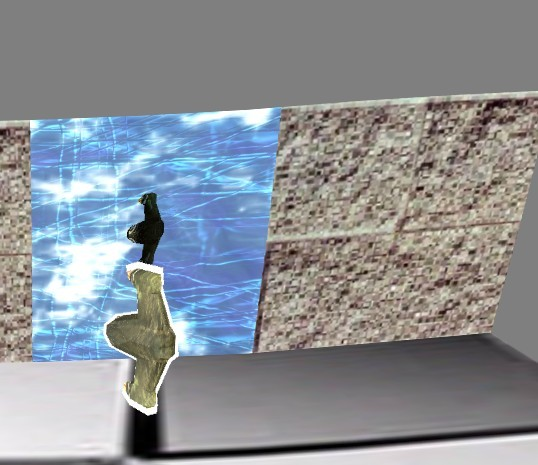[cc lang=”actionscript3″]
private function renderPostProcessing() : void
{
// Render the scene to the scene texture
m_context.setRenderToTexture(m_sceneTexture, true);
renderCube();
m_context.setRenderToBackBuffer();

m_context.setTextureAt(0, m_sceneTexture);
m_context.clear(0.5,0.5,0.5);

m_modelMatrix.identity();
m_modelMatrix.appendTranslation(0,0,1.21);

m_finalMatrix.identity();
m_finalMatrix.append(m_modelMatrix);
m_finalMatrix.append(m_viewMatrix);
m_finalMatrix.append(m_projMatrix);

m_context.setVertexBufferAt(0, m_sceneVertexBuffer, 0, Context3DVertexBufferFormat.FLOAT_3);
m_context.setVertexBufferAt(1,m_sceneVertexBuffer, 3, Context3DVertexBufferFormat.FLOAT_2);

m_context.setProgramConstantsFromMatrix(Context3DProgramType.VERTEX, 0, m_finalMatrix, true);

m_context.drawTriangles(m_sceneIndexBuffer,0,2);
m_context.setTextureAt(0,null);
m_context.setVertexBufferAt(0,null);
m_context.setVertexBufferAt(1,null);
}
[/cc]

m_context.setRenderToTexture(m_sceneTexture, true);

renderCube();

m_context.setRenderToBackBuffer();

Demo is Here : demo

## 3D世界物体拾取 续

[cc lang=”actionscript3″]
/**
* 包围球射线检测
* param o 射线原点
* param d 射线角度
* param c 圆心
* param r 半径
*/
public static function RaySphereIntersect(o : Vector3D, d : Vector3D, c : Vector3D, r : Number) : Boolean
{
var intersect : Boolean;
var s : Number;
var l : Vector3D = c.subtract(o); //圆心到射线原点的距离
s = d.dotProduct(l); //以射线起点开始,以射线的方向步进和包围球的长度的距离

var ll : Number = l.length * l.length; //以射线的起点为圆心,以和包围球的距离为半径假设一个球形
var rr : Number = r * r;

if(s < 0 && ll > rr) return false; //s < 0 表示目标在射线之后， ll > rr 表示射线不在圆内

var mm : Number = ll – s*s; //圆心垂直于射线方向的长度
if(mm > rr) return false; //和射线构成的三角形不在圆内

var q : Number = Math.sqrt(rr – mm); //圆心垂直于射线方向的点到射线碰撞的圆表面点的距离
var t : Number; //射线起点和圆相交点的距离
if(ll > rr) //当射线在圆外
t = s – q;
else
t = s + q;

return true;
}
[/cc]

[cc lang=”actionscript3″]
private function onClick(e:MouseEvent) : void
{
//将鼠标点击位置偏移视口中央
var sceenX : Number = stage.mouseX;
var sceenY : Number = stage.mouseY;

var viewX : Number = (sceenX * 2 / stage.stageWidth) – 1;
var viewY : Number = (-sceenY * 2 / stage.stageHeight) + 1;

var ray : Ray = new Ray();

//将射线转换到相机所在空间
var viewMat : Matrix3D = m_camera.getViewMatrix().clone();
ray.origin = viewMat.transformVector(new Vector3D(0,0,0));

//将相机反转,相机的平移和旋转总是和世界坐标系相反的
viewMat.invert();
ray.direction = viewMat.deltaTransformVector(new Vector3D(viewX,viewY,1));
ray.direction.normalize();

//取出相机的缩放值
var scale : Vector3D = m_proj.decompose();

//将射线转到目标所在空间
var cubeMesh : CubeMesh;
for each(cubeMesh in m_cubeList)
{

//相交检测
var cubePos : Vector3D = cubeMesh.transform.position;
cubePos.x *= scale.x;
cubePos.y *= scale.y;
cubePos.z *= scale.z;

if(Ray.RaySphereIntersect(ray.origin, ray.direction, cubePos, 2))
trace(“碰撞”, m_cubeList.indexOf(cubeMesh));
}
}
[/cc]

btw: 该来的总会来的,工作开始转向用cocos2d-x来制作手游了. stage3D这块还会继续研究的,只是成为副业了. o(︶︿︶)o 唉 真坑爹.

## 在stage3D中实现模型描边效果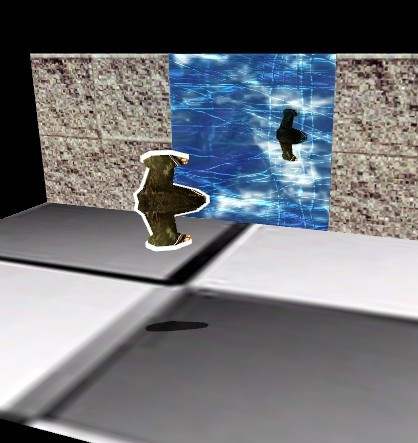Demo 地址：
Demo is here

## 在stage3D中行走在高低起伏的地形之上

[cc lang=”actionscript3″ nowrap=”false”]
public function getHeight(x : Number, z : Number) : Number
[/cc]

[cc lang=”actionscript3″ nowrap=”false”]
x = (m_width >> 1) + x;
z = (m_depth >> 1) – z;
//地形上的x,y. 就是以地形的中心点为前提再偏移. m_width >> 1 和 m_depth >> 1 就是将xz平面的地方 / 2 后,取到中心点.

x /= m_cellSpacing;
z /= m_cellSpacing;

var col : int = Math.floor(x);
var row : int = Math.floor(z);
//我们的地形的每个顶点的间距是 m_cellSpacing ,本demo中的值是10. /= 的方法就是求 col 和 row 的索引值,相信做过2D游戏A*寻路,或者地图区块平铺的同学知道.
[/cc]

[cc lang=”actionscript3″ nowrap=”false”]
var A : Number = getHeightMapEntry(row, col);
var B : Number = getHeightMapEntry(row, col + 1);
var C : Number = getHeightMapEntry(row + 1, col);
var D : Number = getHeightMapEntry(row + 1, col + 1);
[/cc]

return m_heightMap[row * m_numVertsPerRow + col];

[cc lang=”actionscript3″]
var dx : Number = x – col;
var dz : Number = z – row;
//我们将当前位置偏移到这个平面的左上角

var height : Number = 0;
if(dz < 1 - dx) //当 dz < 1-dx 时,表示我们在左上半部分的三角形上 { var uy : Number = B - A; var vy : Number = C - A; height = A + Utils.lerp(0,uy,1 - dx) + Utils.lerp(0,vy,1 - dz); //计算这个三角形邻边和对边的线性插值. Utils.lerp就是计算线性插值的函数. }else{ //当 dz > 1-dx 时,表示我们在右下半部分的三角形上
var uy : Number = C – D;
var vy : Number = B – D;

height = D + Utils.lerp(0,uy,1 – dx) + Utils.lerp(0,vy,1 – dz);
}

return height;
[/cc]

[cc lang=”actionscript3″]
public static function lerp(a : Number, b : Number, t : Number) : Number
{
return a – (a * t) + (b * t);
}
[/cc]

## 在stage3D中创建一个海浪的效果

by the way~ 其实描边什么的我也都实现了,下周开始转向模型了.

## 在stage3D中拾取物体

Sx = Px(Width / 2) + X + Width / 2;

Sy = -Py(Height / 2) + Y + Height / 2;

[00 01 02 03]
[10 11 12 13]
[20 21 22 23]
[30 31 32 33]

[cc lang=”actionscript3″]
package
{
import flash.geom.Vector3D;

public class Ray
{
/**起点**/
public var origin : Vector3D;

/**方向**/
public var direction : Vector3D;

public function Ray()
{
}
}
}
[/cc]

[cc lang=”actionscript3″]
/**
* 计算射线
*/
private function calcPicingRay(x : int, y : int) : Ray
{
var px : Number = 0;
var py : Number = 0;

var vp : Rectangle = new Rectangle(0,0,stage.stageWidth,stage.stageHeight);

var rawData : Vector. = m_proj.rawData;
px = (((2 * x) / vp.width) – 1.0) / rawData;
py = (((-2 * y) / vp.height) + 1.0) / rawData;

var ray : Ray = new Ray();
ray.origin = new Vector3D(0,0,0);
ray.direction = new Vector3D(px,py,1);

return ray;
}
[/cc]

[cc lang=”actionscript3″]
/**
* 转换射线
*/
private function transformRay(ray : Ray, t : Matrix3D) : void
{
//转换射线的原点, w = 1
ray.origin.w = 1;
ray.origin = Utils.subjectMat(ray.origin,t,ray.origin);

//转换射线的方向, w = 0;
ray.direction.w = 0;
ray.direction = Utils.subjectMat(ray.direction,t,ray.direction);

ray.direction.normalize();
}
[/cc]

Utils.subjectMat 是我自己写的向量乘以矩阵的实现函数, 我不知道stage3D中是否有该API,如果有,请告知我是哪个. 这里我也提供下这个函数

[cc lang=”actionscript3″]
//向量左乘矩阵
public static function subjectMat(vec : Vector3D, mat : Matrix3D, out : Vector3D) : Vector3D
{
var data : Vector. = mat.rawData;

if(null == out) out = new Vector3D();

out.x = (vec.x * data + vec.y * data + vec.z * data + vec.w * data);
out.y = (vec.x * data + vec.y * data + vec.z * data + vec.w * data);
out.z = (vec.x * data + vec.y * data + vec.z * data + vec.w * data);
out.w = (vec.x * data + vec.y * data + vec.z * data + vec.w * data);

return out;
}
[/cc]

||P-C|| – R = 0;

|| P0 + tU – C || – R = 0;

At^2 + Bt + C = 0; 这个公式很熟悉吧~ 貌似初中就学过了.
t0 = (-B + Sqrt(B * B – 4AC) / 2;
t1 = (-B – Sqrt(B * B – 4Ac) / 2;

return true;

return false;
}
[/cc]

[cc lang=”actionscript3″]
private function onClick(e:MouseEvent) : void
{
var ray : Ray = calcPicingRay(e.stageX,e.stageY);
var viewInverse : Matrix3D = m_viewMatrix.clone();
viewInverse.invert();
transformRay(ray,viewInverse);

if(raySphereIntTest(ray,m_cubeList)){
tip.text = “碰撞”;
}
else{
tip.text = “W,S,A,D 控制飞船移动n方向键控制相机移动”;
}
}
[/cc]

Demo is Here

## 在stage3D中动态创建贴图并绘制地形阴影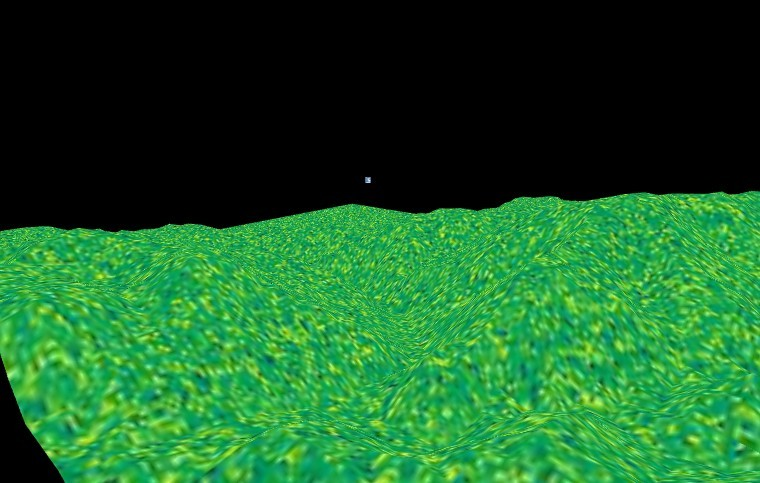[cc lang=”actionscript3″]
public function genTexture(light : Vector3D) : void
{
var texWidth : int = m_numVertsPerRow;
var texHeight : int = m_numVertsPerCol;

var emptyBmd : BitmapData = new BitmapData(texWidth, texHeight,false);
emptyBmd.lock();

for(var i : int = 0; i < texHeight; i ++) { for(var j : int = 0; j < texWidth; j++) { var c : uint; var height : Number = getHeightMapEntry(i,j) / m_heightScale; if(height < 42.5) c = Utils.BEACH_SAND; else if(height < 85) c = Utils.LIGHT_YELLOW_GREEN; else if(height < 127.5) c = Utils.PUREGREEN; else if(height < 170) c = Utils.DARK_YELLOW_GREEN; else if(height < 212.5) c = Utils.DARKBROWN; else c = Utils.WHITE; emptyBmd.setPixel32(j,i,c); } } emptyBmd.unlock(); setTexture(emptyBmd); } public function getHeightMapEntry(row : int, col : int) : int { return m_heightMap[row * m_numVertsPerRow + col]; } public function setTexture(data : BitmapData) : void { m_texture = Utils.getTextureByBmd(data, m_context3D); } [/cc] 当创建好这张动态贴图并贴上之后,就是下图的样子了.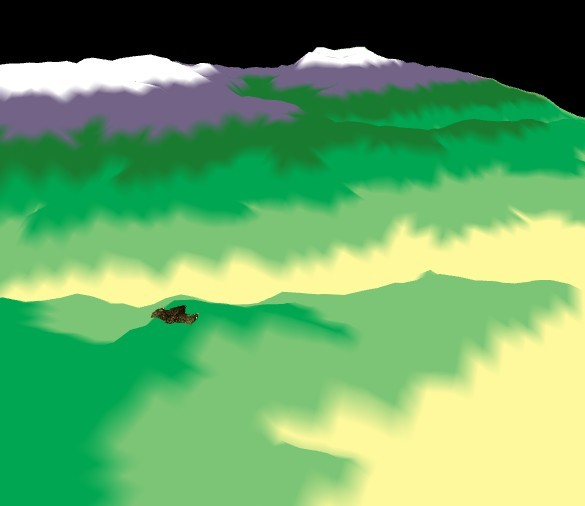## 在stage3D中使用高度图来绘制地形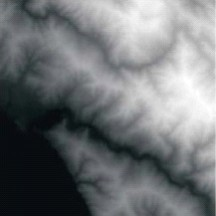[cc lang=”actionscript3″]
[Embed(source=”../../source/coastMountain64.raw”,mimeType = “application/octet-stream”)]
private var terrainData : Class;
[/cc]

[cc lang=”actionscript3″]
private function parseHeightMaps(data : ByteArray) : Boolean
{
if(null == data || 0 == data.bytesAvailable) return false;

var len : uint = data.bytesAvailable;
for(var i : int = 0; i < len; i++){ m_heightMap.push(data.readUnsignedByte()); } return true; } [/cc] 现在我们有了地形的数据了,为了让其显示出来,我们开始创建顶点和索引 顶点的格式是 [x,y,z,u,v] 5个数据 如果每个顶点之间是紧密排列的话,其中u,v的递增量可以通过下面的方式计算出. [cc lang="actionscript3"] var uCoordIncrementSize : Number = 1.0 / 每列顶点数; var vCoordIncrementSize : Number = 1.0 / 每行顶点数; [/cc] 由于高度图是 64 * 64 的大小,我们做 64 * 64的循环来取顶点 startZ = 0, endZ = 63; startX = 0, endX = 63; 如果顶点间是紧密排列的. 那么 m_cellSpacing 为1.0 [cc lang="actionscript3"] for(z = startZ; z >= endZ; z -= m_cellSpacing)
{
j = 0;
for(x = startX; x <= endX; x += m_cellSpacing) { //计算当前顶点缓冲的索引，避免死循环 var index : int = i * m_numVertsPerRow + j; m_rawVertex.push(x,m_heightMap[index],z,j * uCoordIncrementSize,i * vCoordIncrementSize); j++; } i++; } [/cc] 解析完顶点后,就要开始计算索引了.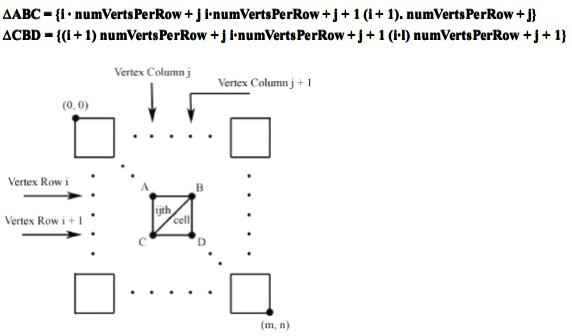[cc lang=”actionscript3″]
private function computeIndices() : void
{
var baseIndex : int = 0;

for(var i : int = 0; i < m_numCellsperCol; i++) { for(var j : int = 0; j < m_numCellsPerRow; j++) { m_rawIndex[baseIndex] = i * m_numVertsPerRow + j; m_rawIndex[baseIndex + 1] = i * m_numVertsPerRow + j + 1; m_rawIndex[baseIndex + 2] = (i + 1) * m_numVertsPerRow + j; m_rawIndex[baseIndex + 3] = (i + 1) * m_numVertsPerRow + j; m_rawIndex[baseIndex + 4] = i * m_numVertsPerRow + j + 1; m_rawIndex[baseIndex + 5] = (i + 1) * m_numVertsPerRow + j + 1; baseIndex += 6; } } trace("索引解析完毕"); } [/cc] 现在我们有了顶点,也有了索引.就可以将其显示出来了. 贴图什么随便设定就好了. demo is here